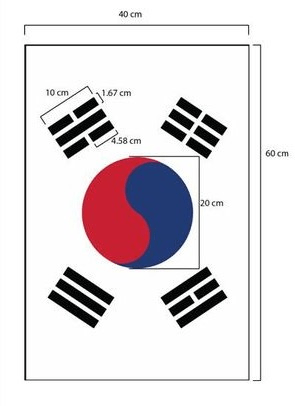Grab Bag
Formulas
Perimeter
Area
Real World
100

The sum of the three angles of any triangle is equal to ______ degrees.

180

100

Formula for area of a parallelogram

A = lw OR A = bh

100

Find the perimeter of the rectangle with a length of 8cm and a width of 5 cm.

P = 26cm

100

Find the area of a rectangle that has a length of 6cm and a width of 5 cm.

A = 30 cm2

100

My family is putting in a new, circular swimming pool. The diameter of the pool is 20 feet. What is the perimeter of our new pool?

62.83 feet

200

Define circumference.

The distance around a circle

200

Formula for area of a square

A = s * s or A = s2

200

Find the perimeter of a square that has a side length of 12cm.

P = 48 cm

200

Find the area of a square with a side length of 7 in.

A = 49 in2

200

Random Trivia: Carrots were not always orange. They were once exclusively __________

A. green

B. purple

C. yellow

purple

300
Write all the factors of 12.
1, 2, 3, 4, 6, 12
300

Formula for area of a triangle

A = 1/2 bh

300

The perimeter of a parallelogram is 28 inches. One pair of sides is 10 inches long. How long is the other pair of sides?

4 inches

300
Find the area of a right triangle with base = 7 cm and height = 8cm.
A = 28 in2
300

Mary needs to fence around her backyard. The length of her backyard is 5m and the width is 7m. If fencing comes in sections of 3m and each section costs $4, how much money will Mary spend?$32.00

400

A parallelogram has an area of 24 units2. Name three possible dimensions of the parallelogram's sides.

6 x 4

8 x 3

12 x 2

24 x 1

400

Random Trivia: How many elections did Abraham Lincoln lose before he became president of the U.S.?

A. 1

B. 3

C. 5

5

400

Random Trivia: Which planet is the only planet to spin clockwise?

Venus

400

A circle has a diameter of 12 inches. What is its area?

113.1 in2

400

A square pizza box has an area of 169 in2. What is the length of one side of the pizza box?

13 in.

500

3 3/4 + 2 1/6

5 11/12

500

Formula for perimeter AND area of a circle

C = 2 pi r

Area = pi r^2

500

Find the perimeter of a pentagon with congruent side length if the sides are 9 inches long.

P= 45 inches

500

A triangle's area is 75 inches2. Its height is 25 inches. What is the length of its base?

b = 6 inches

500

How much of the flag is white?Click to zoom